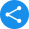# NCERT Solutions for Class 2 Maths

## NCERT Solutions for Class 2 Maths

Latest NCERT solutions Class 2 Maths provided will help students to practice the questions included in class 2 CBSE textbooks. They can study NCERT solutions online with ease through their phones and computers. Studying these books will help them to understand the texts correctly, but solving the questions with the help of NCERT solutions Class 2 Maths is going to assist the young learners in scoring high with ease.

The chapters included in the NCERT class 2 Maths book introduce pupils to basic concepts of mathematics like shapes, skip counting, addition, subtraction, etc. Those who practice these basic concepts thoroughly, along with the solutions, can build a strong foundation in this subject. This makes it easier for them to understand more complex mathematics concepts in higher classes.

Students should opt for Class 2 Maths NCERT solutions to learn according to the latest CBSE standards. It is vital at this stage to practice NCERT Class 2 Maths exercises. It will give them the confidence to ace their tests and score higher marks. Solutions provided here are available for free. Young learners can download them easily without any registration and practice the exercises more effectively. Check out the below-mentioned overview of class 2 CBSE Maths solutions.

Chapter 1: What is Long? What is Round?

This chapter introduces students to different shapes and sizes with the help of fun games and activities. There is also information about types of movement like sliding, rolling, etc. Pupils may download the latest NCERT solutions to practise them properly.

Chapter 2: Counting in Groups

The next chapter in the NCERT textbook is about counting in groups. It teaches students how to quickly calculate the number of objects that are divided into groups. Learners should use free NCERT solution for Class 2 Maths to solve the questions. By the end of this chapter, young learners will be able to count large numbers with ease.

Chapter 3: How Much Can You Carry?

As the name suggests, the chapter is about weights. It uses an exciting story to talk about how things like water can increase and decrease the weight of other objects as well. An important chapter, it is best to solve the exercises according to updated solutions as per the 2019-20 curriculum to prepare for scoring high in exams.

Chapter 4: Counting in Tens

Counting in Tens is a chapter that teaches students about multiplication in tens. It helps them to count objects in groups of ten. Solving the problems in this chapter using the latest NCERT solutions Class 2 Maths will help pupils to score high in exams.

Chapter 5: Patterns

Patterns are an important topic that all readers must learn at a young age. It helps to improve critical thinking through recognition of geometric patterns. Use the CBSE NCERT solutions Class 2 Maths to clear all basic concepts taught in this chapter.

Chapter 6: Footprints

As the book progresses, the chapters become more attractive. Here, students are taught about shapes and sizes through footprints. NCERT Class 2 Maths solutions are available for free download for them to practice.

Chapter 7: Jugs and Mugs

It is another crucial chapter that intuitively teaches about volume and quantity. It begins with instructions to make a lemon drink and then proceeds to show interesting stories that will help students understand the chapters in depth.

Chapter 8: Tens and Ones

This chapter continues to explore the process of counting through ten and ones. Complete the exercises included to improve a student’s understanding of quick counting. Make use of the PDF free download solutions to practice the exercises at home.

Chapter 9: My Funday

Learning about the days in a week has been made fun and intuitive in this chapter. Solving the problems included here will improve overall understanding of students. Latest NCERT solutions Class 2 Maths are provided in PDF format so that they can check answers on their own after solving all exercises.

Chapter 10: Add Our Points

This chapter begins with animals playing in a forest, and learners are asked to add up the points. They can practice addition in an interactive way that lets them have fun in this process as well. Use the latest NCERT solutions Class 2 Maths for easier and better practice of this chapter.

Chapter 11: Lines and Lines

As the chapter name suggests, it is about lines. Students learn about straight and curved lines and how to use them in their everyday life. NCERT textbook solution for Class 2 Maths is provided so that student can practice for better understanding of this chapter’s concepts.

Chapter 12: Give and Take

Here, pupils will learn about addition and subtraction through give and take of objects. They should use the latest NCERT solutions Class 2 Maths to learn about the chapter with confidence.

Chapter 13: The Longest Step

In this chapter, students will learn about different ways and units in which distance is measured. They can use the latest NCERT solutions Class 2 Maths to practice its exercises.

Chapter 14: Birds Come, Birds Go

This chapter provides young learners with a composite idea of counting and addition simultaneously. It is vital that CBSE board students use textbook solutions and practice the problems included in it for proper preparation of exams.

Chapter 15: How Many Ponytails?

The last chapter is a continuation of counting, addition, and easy multiplication through practical ways like counting ponytails, fruits, etc. Free NCERT solution for Class 2 Maths is available that students must use for practice of the same.

### NCERT Solutions for Class 2 Maths

Share this with your friendsShare